## 『统计学』第三部分：假设检验_零假设怎么判断是否有关-程序员宅基地

##### 『假设检验和p值』

• 1. 要解决的问题是什么？
• 2. 证据是什么？
• 3. 判断标准是什么？
• 4. 做出结论

• 首先，第 1 步，明确要解决的问题是什么？

• 第 2 步，证据是什么？

• 第 3 步，判断标准是什么？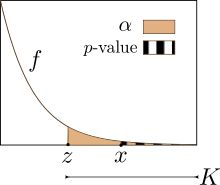• 第 4 步，做出结论

• 要解决的问题是什么？
• 提出零假设和备择假设；
• 证据是什么？
• 假设零假设成立的前提下，得到样本均值的概率 p-值；
• 判断标准是什么？
• 显著性水平 α \alpha （1%, 5%）；
• 做出结论
• p ≤ α p ≤ \alpha ，拒绝零假设，接收备择假设；
• p &gt; α p &gt; \alpha ，接收零假设；

##### 『举个例子』

（1）第 1 步，我们建立两个假设：

H 0 H_0 ：药物对反应时间无影响；（即使使用了药物，反应时间仍是 μ = 1.2 s \mu = 1.2s
H 1 H_1 ：药物有效果；（使用药物后，反应时间 μ = ̸ 1.2 s \mu = \not 1.2s

（2）第 2 步，计算样本发生的概率：

σ ^ X ‾ = 0.05 \hat \sigma_{\overline X} = 0.05

z = 1.05 − 1.2 0.05 = − 3 z = {1.05 - 1.2 \over 0.05} = -3（3）第 3 步，设定一个判断标准：

（4）第 4 步，做出结论：

##### 『单侧检验和双侧检验』

H 0 H_0 ：药物对反应时间无影响；（ μ = 1.2 s \mu = 1.2s
H 1 H_1 ：药物降低反应时间；（使用药物后，反应时间 μ &lt; 1.2 s \mu &lt; 1.2s

##### 『z统计量 VS t统计量』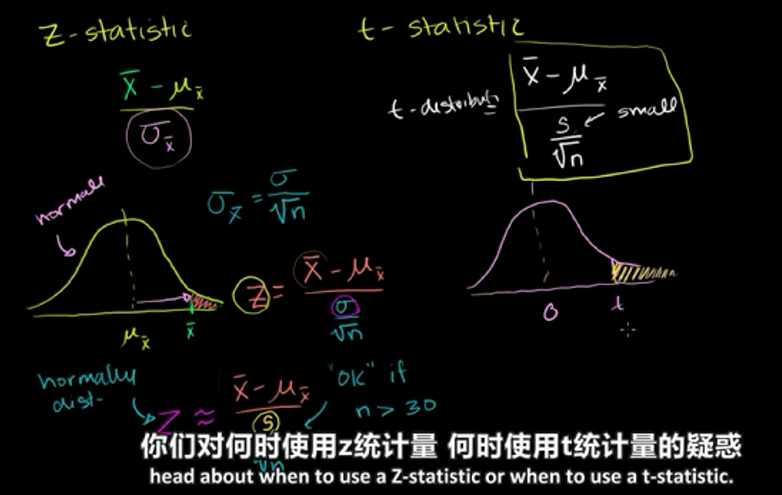X ‾ − μ i σ X ‾ {\overline X - \mu_i \over \sigma_{\overline X}}

z = X ‾ − μ i σ n z = {\overline X - \mu_i \over {\sigma \over \sqrt{n}}}

z ≈ X ‾ − μ i S n z \approx {\overline X - \mu_i \over {S \over \sqrt{n}}}

##### 『小样本假设检验』

X ‾ = 17.17 \overline X = 17.17

S = 2.98 S=2.98

（1）第1步，先定义零假设和备择假设：

H 0 H_0 ：不满足标准，即新引擎的均值正好是百万分之20， μ = 20 p p m \mu=20ppm
H 1 H_1 μ &lt; 20 p p m \mu &lt; 20ppm

（2）第 2 步，计算样本发生的概率：

t = 17.17 − 20 2.98 10 = − 3.0 t = {17.17 - 20 \over {2.98 \over \sqrt{10}}} = -3.0

• 由统计量从z分数表 / t分数表中读出样本发生的概率，然后同判断标准（置信水平）进行比较；
• 通过置信水平求出拒绝区域，然后看z统计量 / t统计量是否落在拒绝域中；

（3）第 3 步，设定一个判断标准：

（4）第 4 步，做出结论：

t统计量的分布是标准化的t分布，如下：##### 『t统计量的置信区间』

− 2.262 &lt; t &lt; 2.262 -2.262 &lt; t &lt; 2.262

− 2.262 &lt; 17.17 − μ 2.98 10 &lt; 2.262 -2.262 &lt; {17.17 - \mu \over {2.98 \over \sqrt{10}}} &lt; 2.262

15.04 &lt; μ &lt; 19.3 15.04 &lt; \mu &lt; 19.3

##### 『大样本占比假设检验』

（1）第 1 步，首先要设定零假设和备择假设：

H 0 ： H_0： 美国家庭总体的互联网接入率小于等于30%；
H 1 ： H_1： 备择假设要与检验的一致，即接入率大于30%，也就是超过30%的美国家庭拥有互联网接入；

（2）第 2 步，计算样本发生的概率：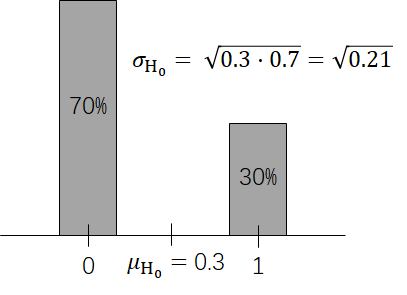σ p ‾ = σ H 0 150 = 0.21 150 = 0.037 \sigma_{\overline p} = {\sigma_{H_0} \over \sqrt{150}} = {\sqrt{0.21} \over \sqrt{150}} = 0.037

z = p ‾ − μ p ‾ σ p ‾ = 0.38 − 0.3 0.037 = 2.14 z = {\overline p - \mu_{\overline p} \over \sigma_{\overline p}} = {0.38 - 0.3 \over 0.037} = 2.14

（3）第 3 步，设定一个判断标准：

（4）第 4 步，做出结论：##### 『随机变量之差的期望和方差』

Z = X + Y Z = X + Y

A = X − Y A = X - Y

E ( Z ) = E ( X ) + E ( Y ) μ z = μ x + μ y E(Z) = E(X) + E(Y) \quad \mu_z =\mu_x + \mu_y

E ( A ) = E ( X ) − E ( Y ) μ A = μ x − μ y E(A) = E(X) - E(Y) \quad \mu_A =\mu_x - \mu_y

σ Z 2 = σ X + Y 2 = σ X 2 + σ Y 2 \sigma_Z^2 = \sigma^2_{X+Y} = \sigma^2_X + \sigma^2_Y

σ A 2 = σ X − Y 2 = σ X 2 + σ Y 2 \sigma_A^2 = \sigma^2_{X-Y} = \sigma^2_X + \sigma^2_Y

##### 『样本均值之差的分布』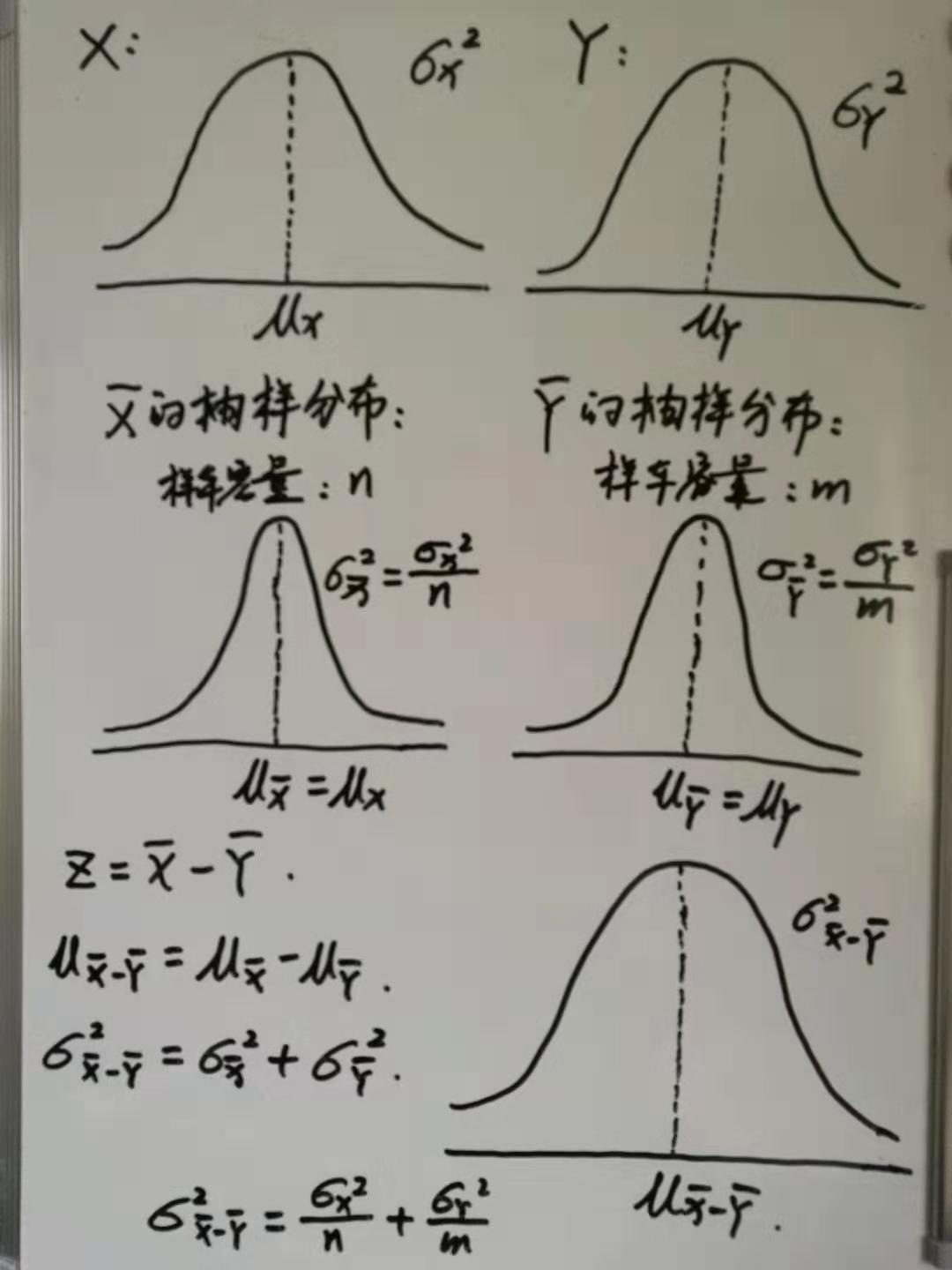Z是一个随机变量，从X的总体中取n个样本求均值，从Y的总体中取m个样本求均值，然后再求出两均值之差，这就是随机变量Z，那么Z的分布是什么样的呢？

μ X ‾ − Y ‾ = μ X ‾ − μ Y ‾ \mu_{\overline X - \overline Y} = \mu_{\overline X} - \mu_{\overline Y}

σ X ‾ − Y ‾ 2 = σ X ‾ 2 + σ Y ‾ 2 = σ X 2 n + σ Y 2 m \sigma^2_{\overline X - \overline Y} = \sigma^2_{\overline X} + \sigma^2_{\overline Y} = {\sigma^2_{X} \over n} + {\sigma^2_{Y} \over m}

##### 『样本均值之差的置信区间』σ X ‾ 1 − X ‾ 2 2 = σ X ‾ 1 2 + σ X ‾ 2 2 = σ X 1 2 100 + σ X 2 2 100 = S 1 2 100 + S 2 2 100 \sigma^2_{\overline X_1 - \overline X_2} = \sigma^2_{\overline X_1} + \sigma^2_{\overline X_2} = {\sigma^2_{X_1} \over 100} + {\sigma^2_{X_2} \over 100} = {S^2_1 \over 100} + {S^2_2 \over 100}

σ X ‾ 1 − X ‾ 2 = 0.617 \sigma_{\overline X_1 - \overline X_2} = 0.617

1.96 ∗ σ X ‾ 1 − X ‾ 2 = 1.21 1.96 * \sigma_{\overline X_1 - \overline X_2} = 1.21

μ X ‾ − Y ‾ = μ X ‾ − μ Y ‾ = μ 1 − μ 2 \mu_{\overline X - \overline Y} = \mu_{\overline X} - \mu_{\overline Y} = \mu_1 - \mu_2

##### 『样本均值之差的假设检验』

H 0 ： H_0： 低脂节食没有作用，即： μ 1 − μ 2 = 0 ⇒ μ X ‾ 1 − μ X ‾ 2 = 0 ⇒ μ X ‾ 1 − X ‾ 2 = 0 \mu_1 - \mu_2 = 0 \Rightarrow \mu_{\overline X_1} - \mu_{\overline X_2} = 0 \Rightarrow \mu_{\overline X_1 - \overline X_2} = 0
H 1 ： H_1： 低脂节食有效，即： μ 1 − μ 2 &gt; 0 ⇒ μ X ‾ 1 − X ‾ 2 &gt; 0 \mu_1 - \mu_2 &gt; 0 \Rightarrow \mu_{\overline X_1 - \overline X_2} &gt; 0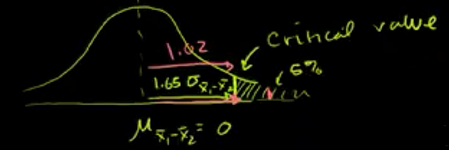##### 『总体占比的比较』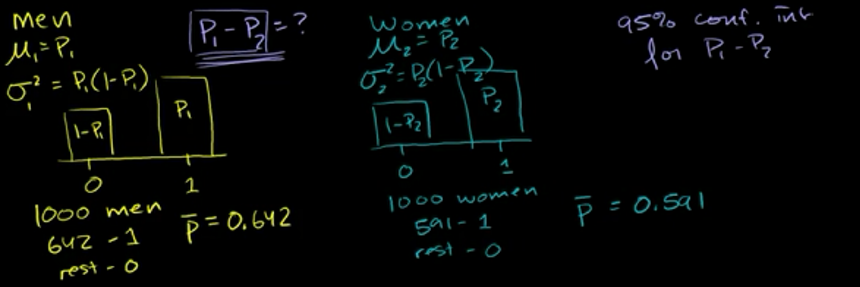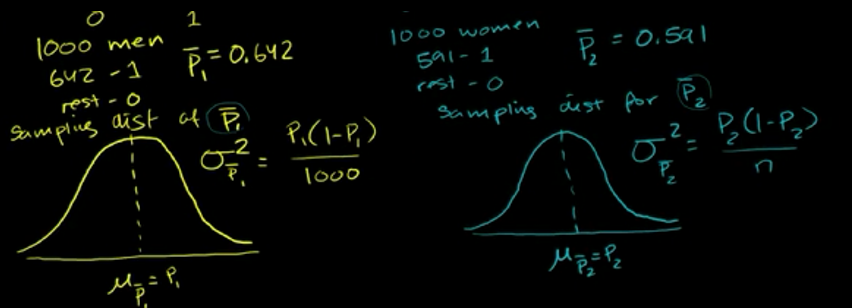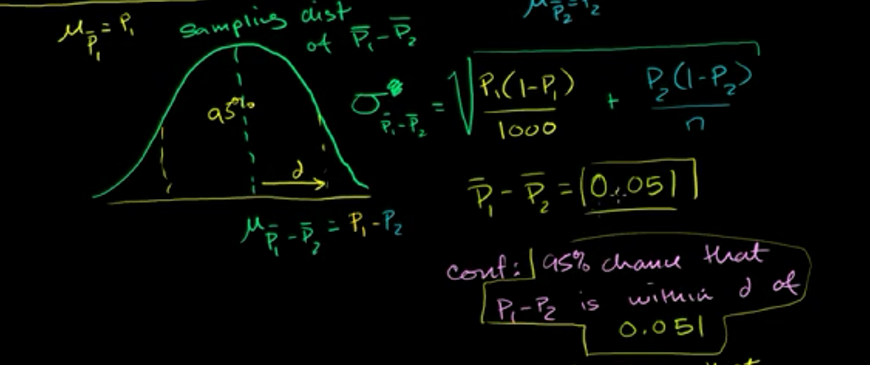σ p 1 − p 2 = 0.022 \sigma_{p_1 - p_2} = 0.022

d = 1.96 ∗ σ p 1 − p 2 = 0.043 d = 1.96 * \sigma_{p_1 - p_2} = 0.043

##### 『总体占比的比较的假设检验』

H 0 ： H_0： 男女投票占比无差别， p 1 = p 2 ， 即 p 1 − p 2 = 0 p_1 = p_2，即 p_1 - p_2 = 0
H 1 ： H_1： 男女投票占比存在差异， p 1 = ̸ p 2 ， 即 p 1 − p 2 = ̸ 0 p_1 = \not p_2，即 p_1 - p_2 = \not 0

z = 0.051 − 0 σ p ‾ 1 − p ‾ 2 z = {0.051 - 0 \over \sigma_{\overline p_1 - \overline p_2}}

z = 0.051 − 0 0.0217 = 2.35 z = {0.051 - 0 \over 0.0217} = 2.35

 知乎：假设检验的逻辑是是什么？
 可汗学院 统计